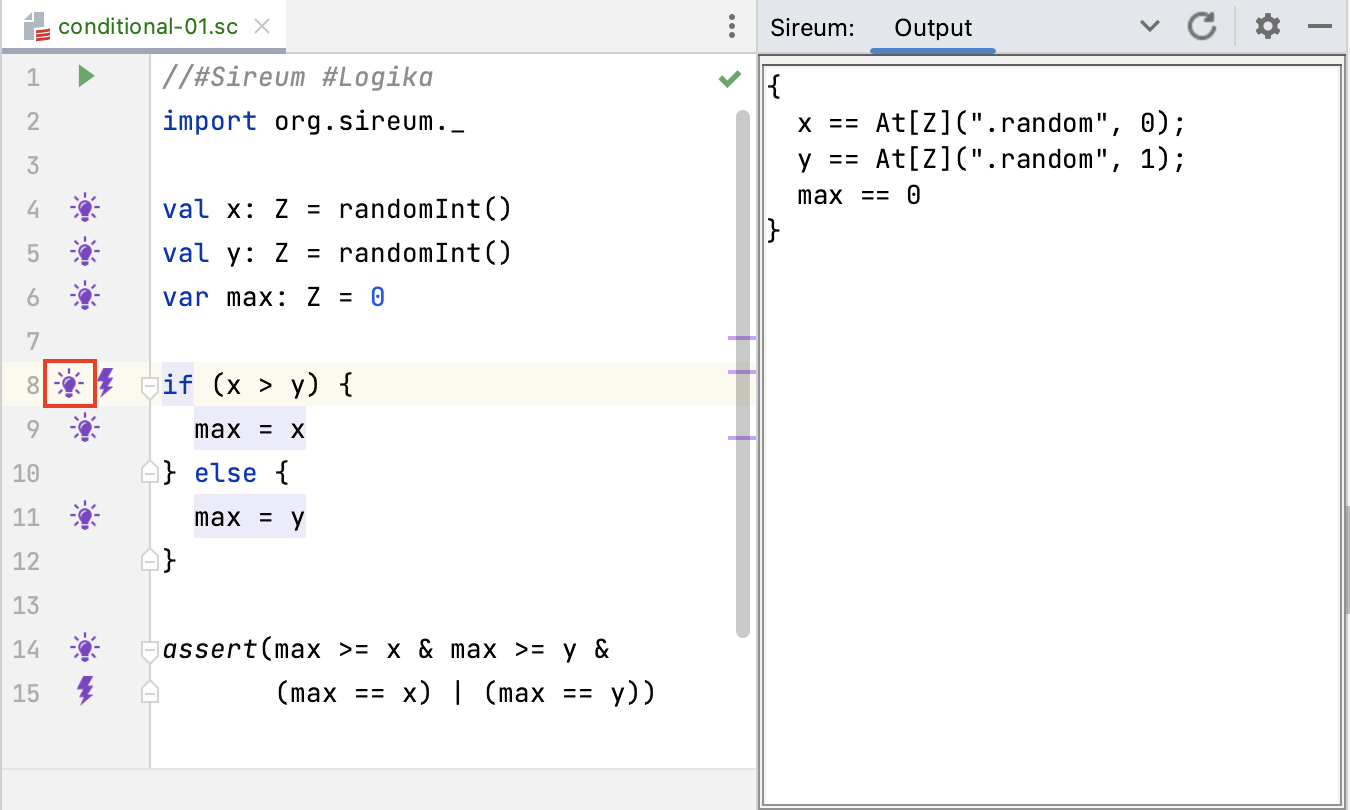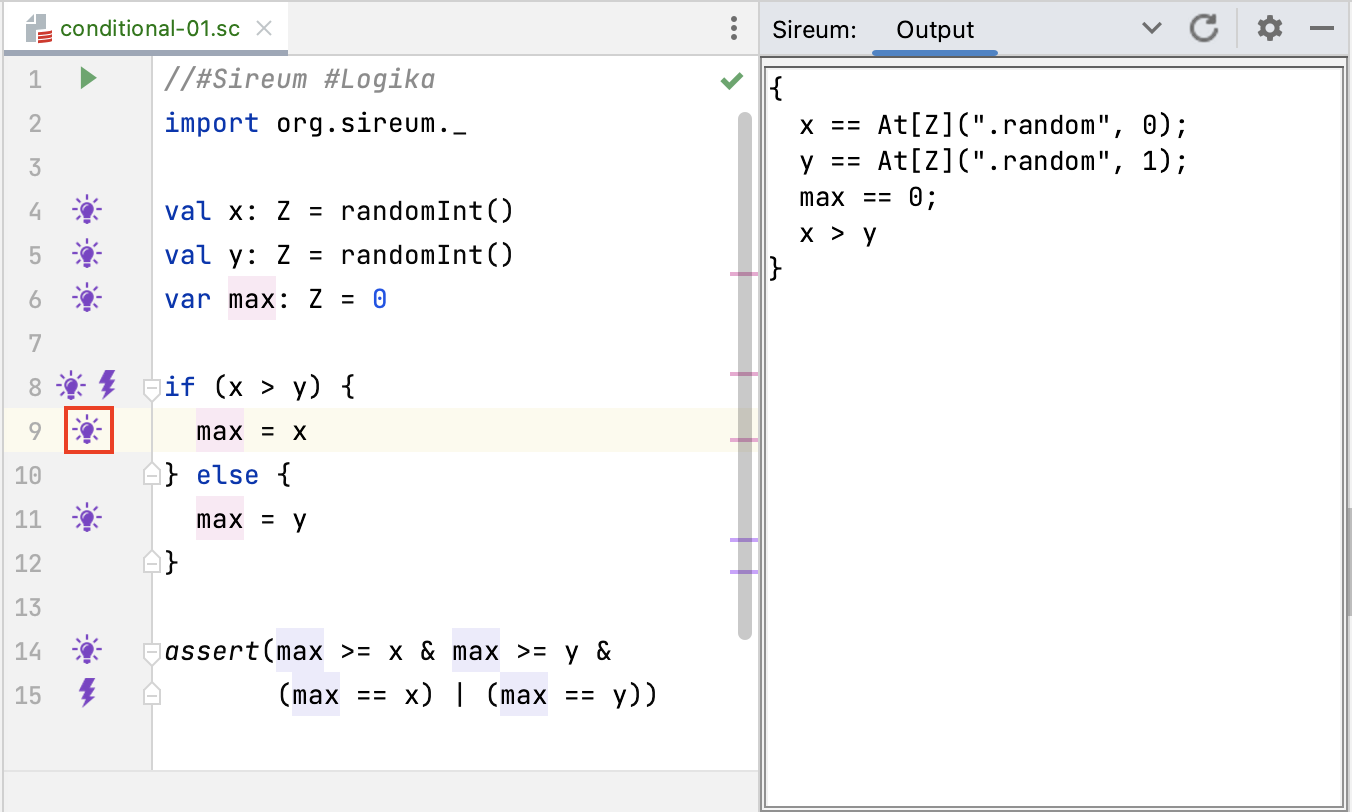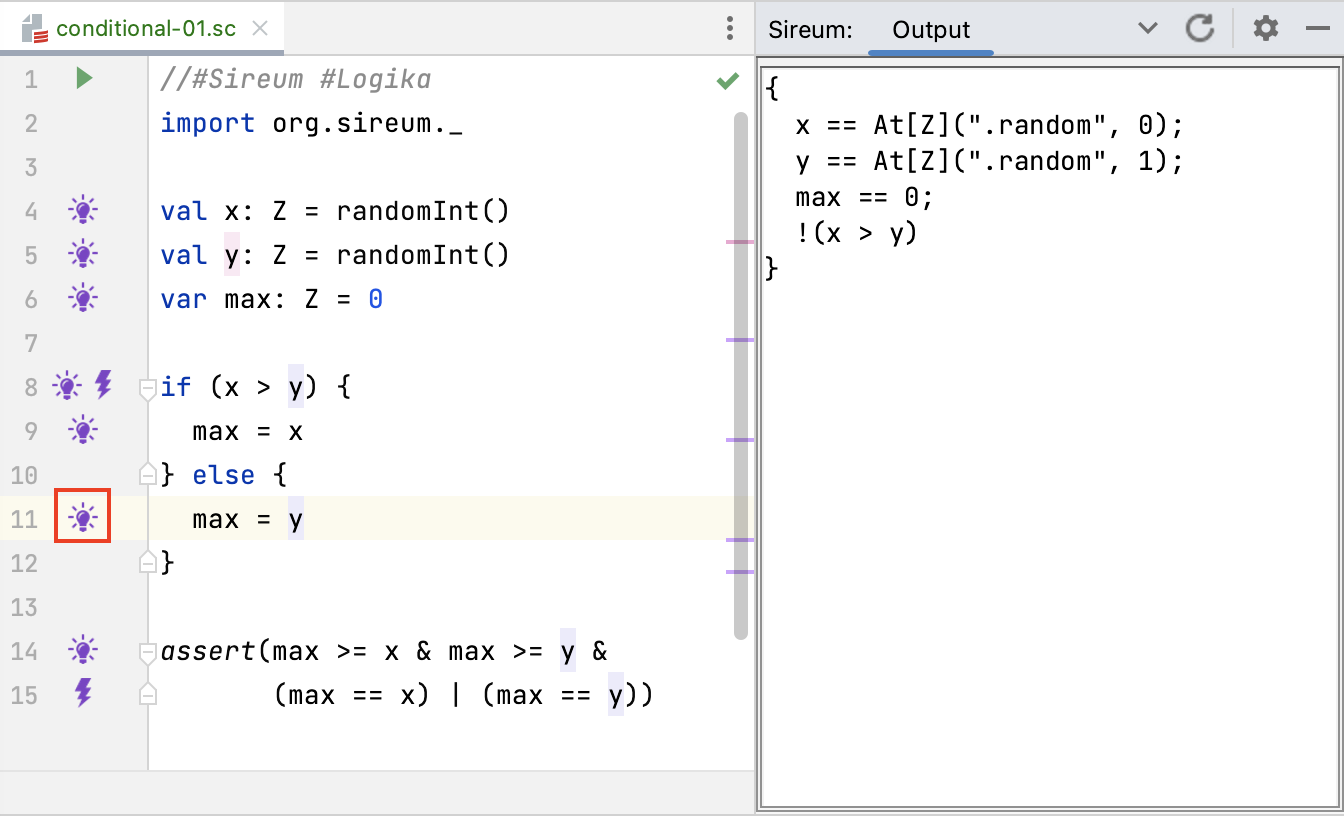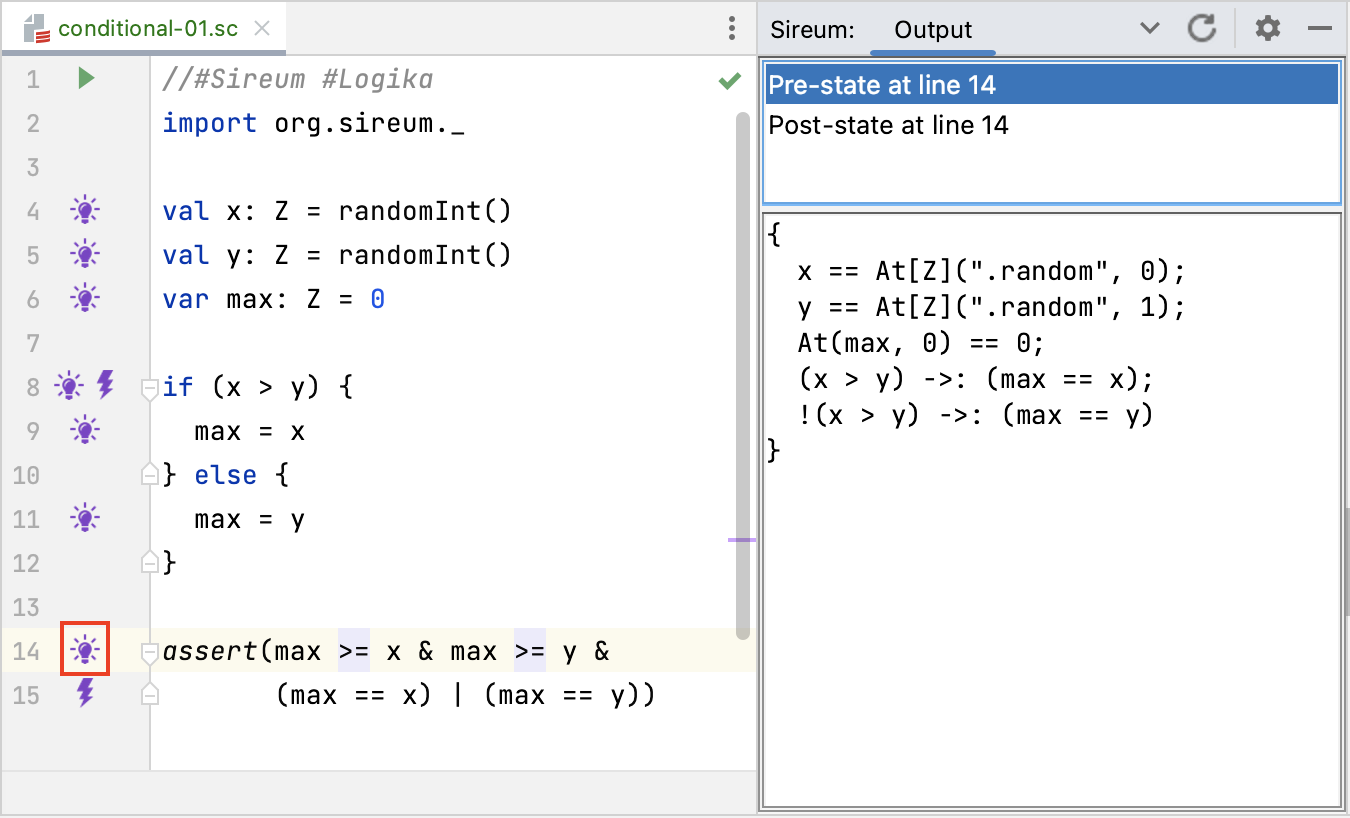## Logika Automated Reasoning for Conditionals

There are three key points to understand about how Logika automated deduction deals with `if` statements:

• checking the test expression: when Logika encounters an `if` statement, it will using a summoning of the SMT solvers to see, based on the known facts at that point, if it can determine if the `if` test expression is true or false. If the summoning identicates that the test expression is true or false, Logika will process the corresponding branch. The more common case is that it cannot determine whether the test expression is true or not, and in this case Logika processes both branches of the `if` statement.
• checking each branch, with expanded knowledge about the test expression: as Logika descends into a branch of a `if`, it will add to its known facts the logical condition that must be true for control to flow into that branch. For example, in the max example above, upon entering the true branch, Logika will record in its known facts `x > y`, i.e., the test expression of the `if` statement holds within the true branch. When entering the false branch, Logika indicates in the known facts that the test expression is false, i.e., `!(x > y)`
• merging knowledge of both branches when the `if` statement is complete: when Logika is unable to decide the test expression and has to explore both true and false branches, at the completion of the `if` Logika must merge facts from both branches while keeping track of which branch the facts apply to. It does this facts that are conditioned on the value of the test expression, e.g., from the true branch, it will keep the fact `(x > y) ->: (max == x)` after the conditional completes.

We now illustrate how this process is reflected in the IVE.

First, consider the known facts at the top of the loop at Line 8 (before the test expression is evaluated).Here, we see only the basic facts about the variables `x`,`y`, and `z`. In particular, since `x` and `y` are unconstrained, Logika is unable to determine if the test expression `x > y` is true or false. Therefore, it will explore both branches (and we can see that since lightbulb annotations appear in both branches at Line 9 and Line 11).

Now consider the known facts as we enter the true branch at Line 9. Since we are inside the true branch, we are dealing with the case where the test expression must be true, and so Logika adds `x > y` to the known facts, as illustrated below.On the other hand, the following screen shot shows the known facts at Line 11 as Logika explores the false branch – `!(x > y)` has been added to the known facts, indicating that the test expression is false.After the conclusion of the `if` statement, the known facts at Line 14 show Logika has merged the knowledge from both branches: the facts originating in each branch of the `if` are now conditioned (using implication `->:`) on the value of the test expression within the respective branch.The aggregated known facts at this point are sufficient for the SMT solvers to prove that assertion at Line 14 hold, which captures the correctness property of the “find max” calculation.